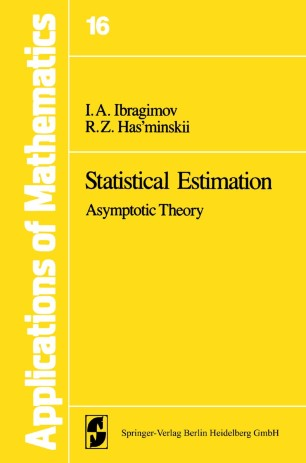# Statistical Estimation

## Asymptotic Theory

• I. A. Ibragimov
• R. Z. Has’minskiiPart of the Applications of Mathematics book series (SMAP, volume 16)

1. Front Matter
Pages i-vii
2. I. A. Ibragimov, R. Z. Has’minskii
Pages 1-2
3. I. A. Ibragimov, R. Z. Has’minskii
Pages 3-9
4. I. A. Ibragimov, R. Z. Has’minskii
Pages 10-112
5. I. A. Ibragimov, R. Z. Has’minskii
Pages 113-172
6. I. A. Ibragimov, R. Z. Has’minskii
Pages 173-213
7. I. A. Ibragimov, R. Z. Has’minskii
Pages 214-240
8. I. A. Ibragimov, R. Z. Has’minskii
Pages 241-280
9. I. A. Ibragimov, R. Z. Has’minskii
Pages 281-320
10. I. A. Ibragimov, R. Z. Has’minskii
Pages 321-361
11. Back Matter
Pages 363-403

### Introduction

when certain parameters in the problem tend to limiting values (for example, when the sample size increases indefinitely, the intensity of the noise ap­ proaches zero, etc.) To address the problem of asymptotically optimal estimators consider the following important case. Let X 1, X 2, ... , X n be independent observations with the joint probability density !(x,O) (with respect to the Lebesgue measure on the real line) which depends on the unknown patameter o e 9 c R1. It is required to derive the best (asymptotically) estimator 0:( X b ... , X n) of the parameter O. The first question which arises in connection with this problem is how to compare different estimators or, equivalently, how to assess their quality, in terms of the mean square deviation from the parameter or perhaps in some other way. The presently accepted approach to this problem, resulting from A. Wald's contributions, is as follows: introduce a nonnegative function w(0l> ( ), Ob Oe 9 (the loss function) and given two estimators Of and O! n 2 2 the estimator for which the expected loss (risk) Eown(Oj, 0), j = 1 or 2, is smallest is called the better with respect to Wn at point 0 (here EoO is the expectation evaluated under the assumption that the true value of the parameter is 0). Obviously, such a method of comparison is not without its defects.

### Keywords

Asymptotische Wirksamkeit Estimation Theory Estimator Parameter Schätzung (Statistik)

#### Authors and affiliations

• I. A. Ibragimov
• 1
• R. Z. Has’minskii
• 2